# How to set the Visibility of MaskedTextBox in C#?

In C#, MaskedTextBox control gives a validation procedure for the user input on the form like date, phone numbers, etc. Or in other words, it is used to provide a mask which differentiates between proper and improper user input. In MaskedTextBox control, you are allowed to set the visibility of the MaskedTextBox using Visible Property provided by the MaskedTextBox control.
If the value of this property is set to true, then the MaskedTextBox control and its child control are visible on the screen. And if the value of this property is set to false, then the MaskedTextBox control and its child control are not visible on the screen. You can set this property in two different ways:

1. Design-Time: It is the easiest way to set the value of Visible property of MaskedTextBox control as shown in the following steps:

• Step 1: Create a windows form as shown in the below image:

Visual Studio -> File -> New -> Project -> WindowsFormApp• Step 2: Next, drag and drop the MaskedTextBox control from the toolbox on the form as shown in the below image: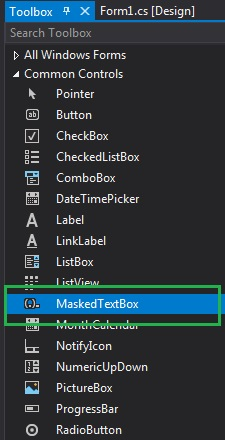• Step 3: After drag and drop you will go to the properties of the MaskedTextBox and set the value of Visible property of MaskedTextBox control as shown in the below image: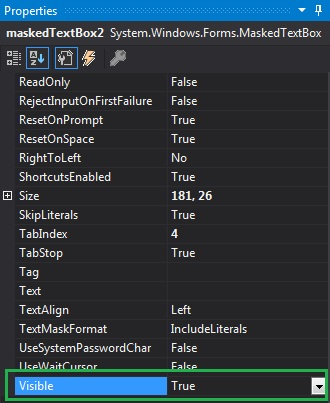Output: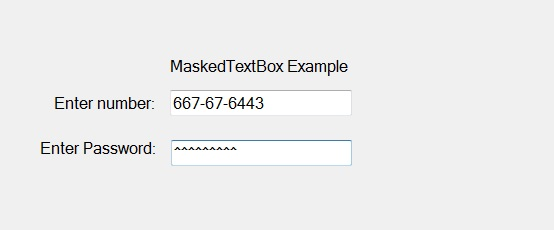2. Run-Time: It is a little bit trickier than the above method. In this method, you can set the visibility of the MaskedTextBox control programmatically with the help of given syntax:

`public bool Visible { get; set; }`

The value of this property is of System.Boolean type, either true or false. The following steps show how to set the visibility of the MaskedTextBox control dynamically:

```// Creating a MaskedTextBox
```
• Step 2: After creating MaskedTextBox, set the Visible property of the MaskedTextBox provided by the MaskedTextBox class.
```// Setting the visibility
m.Visible = false;
```
• Step 3: And last add this MaskedTextBox control to the form using the following statement:
```// Adding MaskedTextBox
// control on the form
```

Example:

 `using` `System; ` `using` `System.Collections.Generic; ` `using` `System.ComponentModel; ` `using` `System.Data; ` `using` `System.Drawing; ` `using` `System.Linq; ` `using` `System.Text; ` `using` `System.Threading.Tasks; ` `using` `System.Windows.Forms; ` ` `  `namespace` `WindowsFormsApp39 { ` ` `  `public` `partial` `class` `Form1 : Form { ` ` `  `    ``public` `Form1() ` `    ``{ ` `        ``InitializeComponent(); ` `    ``} ` ` `  `    ``private` `void` `Form1_Load(``object` `sender, EventArgs e) ` `    ``{ ` ` `  `        ``// Creating and setting the ` `        ``// properties of the Label ` `        ``Label l1 = ``new` `Label(); ` `        ``l1.Location = ``new` `Point(413, 98); ` `        ``l1.Size = ``new` `Size(176, 20); ` `        ``l1.Text = ``" Example"``; ` `        ``l1.Font = ``new` `Font(``"Bell MT"``, 12); ` ` `  `        ``// Adding label on the form ` `        ``this``.Controls.Add(l1); ` ` `  `        ``// Creating and setting the ` `        ``// properties of the Label ` `        ``Label l2 = ``new` `Label(); ` `        ``l2.Location = ``new` `Point(242, 135); ` `        ``l2.Size = ``new` `Size(126, 20); ` `        ``l2.Text = ``"Phone number:"``; ` `        ``l2.Font = ``new` `Font(``"Bell MT"``, 12); ` ` `  `        ``// Adding label on the form ` `        ``this``.Controls.Add(l2); ` ` `  `        ``// Creating and setting the  ` `        ``// properties of MaskedTextBox ` `        ``MaskedTextBox m = ``new` `MaskedTextBox(); ` `        ``m.Location = ``new` `Point(374, 137); ` `        ``m.Mask = ``"000000000"``; ` `        ``m.Size = ``new` `Size(176, 20); ` `        ``m.Name = ``"MyBox"``; ` `        ``m.BorderStyle = BorderStyle.Fixed3D; ` `        ``m.Visible = ``false``; ` `        ``m.Font = ``new` `Font(``"Bell MT"``, 18); ` ` `  `        ``// Adding MaskedTextBox  ` `        ``// control on the form ` `        ``this``.Controls.Add(m); ` `    ``} ` `} ` `} `

Output:

Before setting the value of Visible property to false: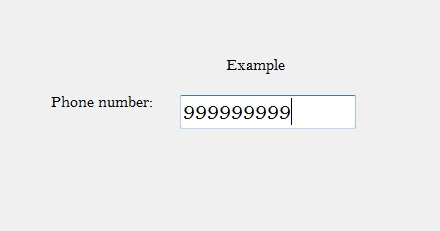After setting the value of Visible property to false: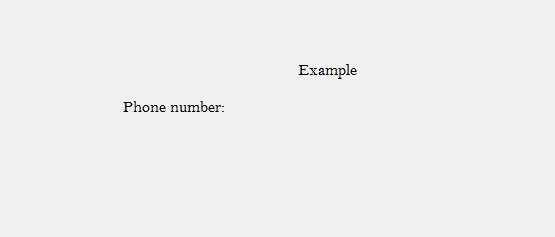My Personal Notes arrow_drop_upCheck out this Author's contributed articles.

If you like GeeksforGeeks and would like to contribute, you can also write an article using contribute.geeksforgeeks.org or mail your article to contribute@geeksforgeeks.org. See your article appearing on the GeeksforGeeks main page and help other Geeks.

Please Improve this article if you find anything incorrect by clicking on the "Improve Article" button below.

Article Tags :

Be the First to upvote.

Please write to us at contribute@geeksforgeeks.org to report any issue with the above content.